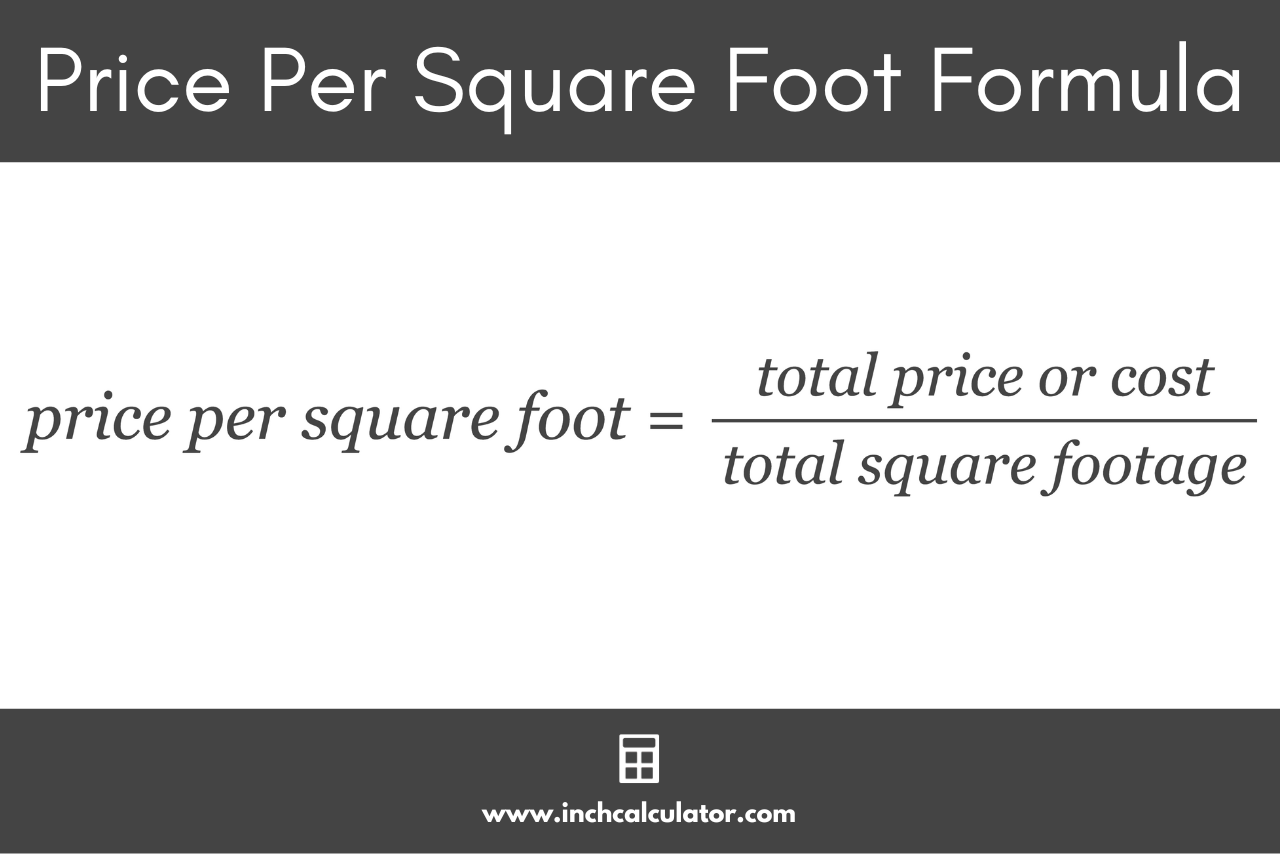# Price Per Square Foot Calculator

Use the calculator below to calculate the price per square foot given the price or rent and the area in square feet, yards, or meters.

\$

## Price Per Square Foot:

-
Learn how we calculated this below

## How to Calculate the Price Per Square Foot

The price per square foot is used for several things, from property purchases to home renovations. It’s useful as a uniform metric to compare and evaluate the cost of projects or the value of properties.

You can calculate the price per square foot using a simple formula.

### Price Per Square Foot Formula

The formula for calculating the price per square foot is straightforward. The formula is:

price per square foot = total price or cost / total square footage

Where:

• total price or cost is the overall price of the property or the total cost of a project.
• total square footage is the total area (in square feet) of the property or project.

So, the price per square foot is equal to the total price divided by the total area in square feet.For example, let’s say you’re interested in buying a home listed for \$300,000. The total area of this house is 2,500 square feet.

To determine the price per square foot, you can divide the total cost of the house (\$300,000) by its total square footage (2,500 sq ft).

price per square foot = \$300,000 / 2,500 sq ft = \$120/sq ft

Thus, the house costs \$120 per square foot.

## Price Per Square Foot Applications

The price per square foot metric is frequently used in various sectors, especially real estate. Here are some of its applications:

• Real Estate: Buyers, sellers, and real estate agents often utilize this metric to compare property values in different neighborhoods or regions. It helps determine if a property is overpriced or a bargain in its respective market and is often used when comparing rental properties.
• Renovations: Homeowners and contractors use the price per square foot to estimate the cost of renovations or home improvements. For instance, understanding the price per square foot of installing hardwood can help homeowners budget appropriately.
• Commercial Leasing: In commercial real estate, rent is sometimes determined based on the price per square foot, especially in retail spaces. It helps businesses gauge the cost-effectiveness of leasing a particular space.
• Construction: When building homes or commercial properties, understanding the cost per square foot can help with budgeting and determining the financial feasibility of a project.

The price per square foot is a commonly used metric whenever a standardized means of assessing value, cost, or comparison is needed.